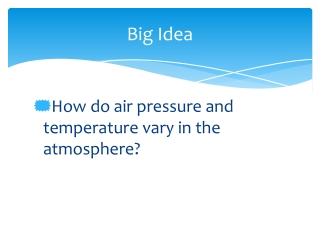DownloadDownload PresentationBig Idea

# Big Idea

Télécharger la présentation## Big Idea

- - - - - - - - - - - - - - - - - - - - - - - - - - - E N D - - - - - - - - - - - - - - - - - - - - - - - - - - -
##### Presentation Transcript

1. Big Idea How do air pressure and temperature vary in the atmosphere?

2. Layers of the Atmosphere Chapter 7, Section 3

3. Key Questions What kinds of questions can you ask based on this picture?

4. Key Questions What kinds of questions related to the atmosphere can you ask?

5. Key Questions SWBAT list the four main layers of the atmosphere SWBAT explain the characteristics of each layer

6. Troposphere The lowest layer of the Earth’s atmosphere. ‘Tropo-’ means ‘turning’ or ‘changing.’ The troposphere is the layer where Earth’s weather occurs.

7. Stratosphere ‘strato’ means ‘layer’ or ‘spread out.’ The stratosphere is the second layer, right above the troposphere. The stratosphere contains the ozone layer.

8. Mesosphere ‘Meso’ means middle. The mesosphere is the middle layer, above the stratosphere and below the thermosphere. The mesosphere protects Earth’s surface from being hit by most meteoroids.

9. Thermosphere ‘Thermo’ means heat. The thermosphere is Earth’s outermost layer, which starts above the mesosphere and extends into space. This is the hottest layer because sunlight strikes this area first.

10. Temperature The average amount of energy of motion of each molecule of a substance. How does temperature change as you go up in the atmosphere?

11. Ionosphere Ions are charged particles. The ionosphere contains charged particles in the air, which creates the aurora borealis effect. The ionosphere is the bottom part of the thermosphere.

12. Exosphere ‘Exo’ means ‘outer.’ The exosphere is the outer portion of the thermosphere

13. Is Air There? • Hypothesis: I think air is/is not in the jar because… • Materials: glass jar, plastic bag, rubber band • Predict: When the plastic bag is secured tightly around the rim of the jar, what do you think will happen in terms of air pressure? • Observe: • Predict: When the plastic bag is secured tightly the opposite way, what do you think will happen in terms of air pressure? • Observe:

14. Lab: Today’s Air Pressure

15. Four main layers of the atmosphere Scientists divide Earth’s atmosphere into four main layers classified according to changes in temperature.

16. Key Questions What are the four main layers of the atmosphere?

17. Key Questions • What are the four main layers of the atmosphere? • Scientists divide Earth’s atmosphere into four main layers classified according to changes in temperature. These layers are the troposphere, the stratosphere, the mesosphere, and the thermosphere.

18. Key Questions • What are the four main layers of the atmosphere? • Scientists divide Earth’s atmosphere into four main layers classified according to changes in temperature. These layers are the troposphere, the stratosphere, the mesosphere, and the thermosphere. • What are the characteristics of each layer?

19. Key Questions • What are the four main layers of the atmosphere? • Scientists divide Earth’s atmosphere into four main layers classified according to changes in temperature. These layers are the troposphere, the stratosphere, the mesosphere, and the thermosphere. • What are the characteristics of each layer? • The troposphere is the layer of the atmosphere in which Earth’s weather occurs.

20. What are the four main layers of the atmosphere? • Scientists divide Earth’s atmosphere into four main layers classified according to changes in temperature. These layers are the troposphere, the stratosphere, the mesosphere, and the thermosphere. • What are the characteristics of each layer? • The troposphere is the layer of the atmosphere in which Earth’s weather occurs. • The stratosphere is the second layer of the atmosphere and contains the ozone layer.

21. What are the four main layers of the atmosphere? • Scientists divide Earth’s atmosphere into four main layers classified according to changes in temperature. These layers are the troposphere, the stratosphere, the mesosphere, and the thermosphere. • What are the characteristics of each layer? • The troposphere is the layer of the atmosphere in which Earth’s weather occurs. • The stratosphere is the second layer of the atmosphere and contains the ozone layer. • The mesosphere is the layer of the atmosphere that protects Earth’s surface from being hit by most meteoroids.

22. What are the four main layers of the atmosphere? • Scientists divide Earth’s atmosphere into four main layers classified according to changes in temperature. These layers are the troposphere, the stratosphere, the mesosphere, and the thermosphere. • What are the characteristics of each layer? • The troposphere is the layer of the atmosphere in which Earth’s weather occurs. • The stratosphere is the second layer of the atmosphere and contains the ozone layer. • The mesosphere is the layer of the atmosphere that protects Earth’s surface from being hit by most meteoroids. • The outermost layer of Earth’s atmosphere is the thermosphere.

23. Homework Workbook Chapter 7 Section 3 pg. 137 - 140